# Factoring Polynomials

Factoring Polynomials
Go back to  'Polynomials'

You have already studied algebraic identities like $$(a+b)^2=a^2+2ab+b^2$$,$$(a-b)^2=a^2-2ab+b^2$$, and $$(a+b)(a-b)=a^2-b^2$$.

Have you ever wondered how do we get these identities?

Here, is a visual representation of the identity $$(a+b)^2=a^2+2ab+b^2$$.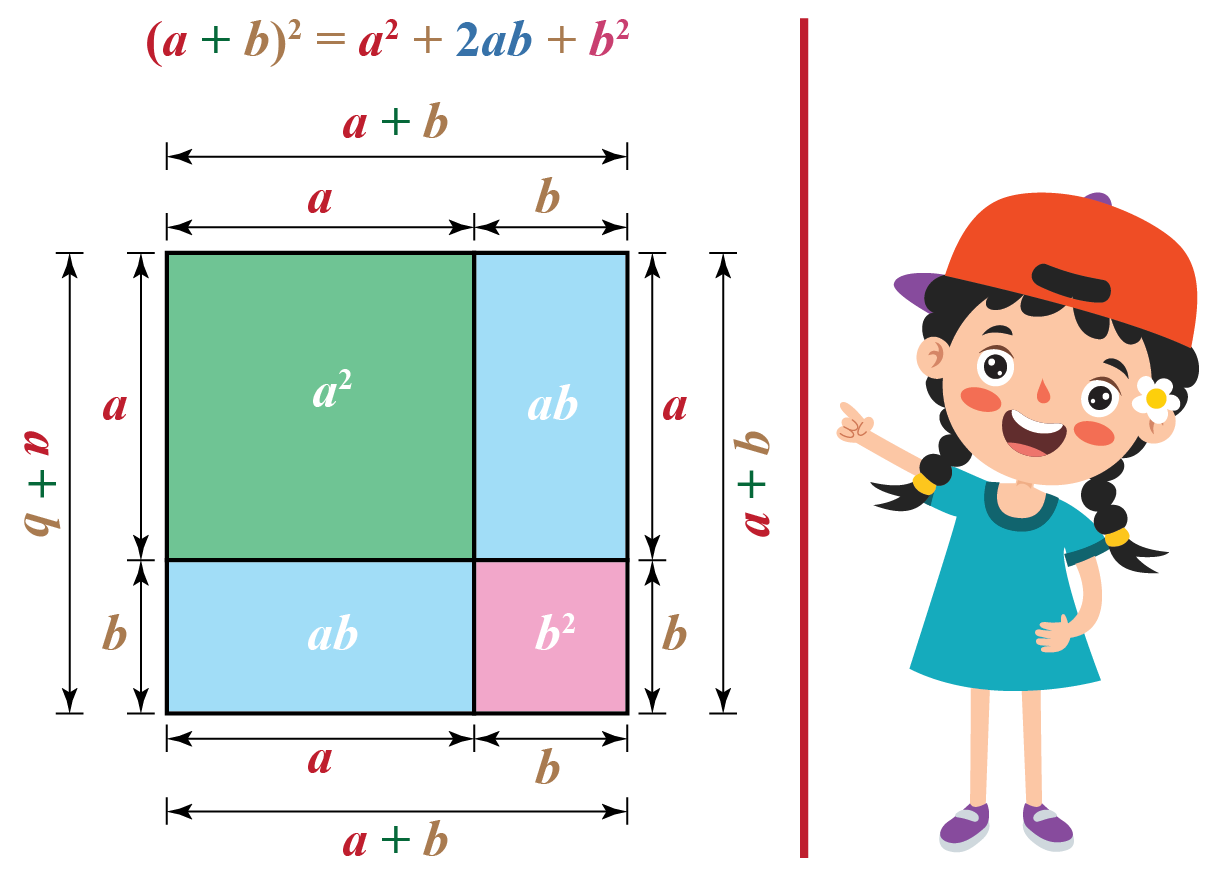This means $$a^2+2ab+b^2$$ can be expressed as the square of linear factors of a polynomial, that is, $$(a+b)^2$$.

In this mini-lesson, we will explore the world of factoring polynomials in math. You will understand factoring polynomials by grouping, factoring cubic polynomials, prime factorization of polynomials, linear factors of a polynomial and other interesting facts around the topic. You can try your hand at solving a few interesting practice questions at the end of the page.

## What Do You Mean By Factorisation of Polynomials?

Expressing a polynomial using the product of its factors is called factorization of polynomials.

Consider, a polynomial $$x^3-3x^2$$.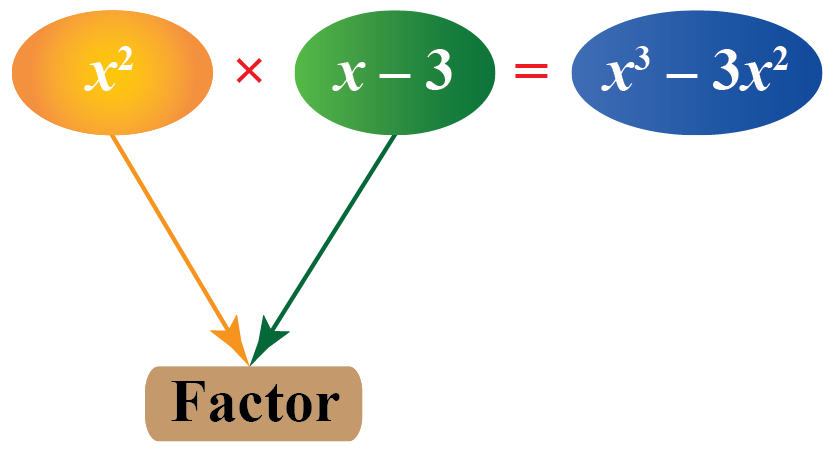Here, $$x^2\times (x-3)$$ is the factorization of $$x^3-3x^2$$.

So, factoring cubic polynomial $$x^3-3x^2$$ gives $$x^3-3x^2=x^2(x-3)$$

## How To Factorize Polynomials?

### Steps

Follow the steps mentioned below to factorize a polynomial.

1. Factor out if there is a factor common to all the terms of the polynomial.
2. Identify the appropriate method to factorize the polynomial. You can use regrouping or algebraic identities to find the factors of the polynomial.
3. Write polynomial as the product of its factors.

### Examples

Consider a polynomial: $$8ab+8b+28a+28$$

Notice that 4 is a single factor common to all the terms of this polynomial.

So, we can write
$$8ab+8b+28a+28$$
$$=4(2ab+2b+7a+7)$$

Here, we will do factoring polynomial by grouping.

Let us write $$2ab+2b$$ and $$7a+7$$ in the factor form separately.

\begin{align}2ab+2b&=2b(a+1)\\7a+7&=7(a+1)\end{align}

So, $$8ab+8b+28a+28$$
$$=4(2ab+2b+7a+7)$$
$$=4\left(2b(a+1)+7(a+1)\right)$$

Again, we have a common factor $$(a+1)$$ on the right-hand side.

The given expression is factorized as $$8ab+8b+28a+28=4(2b+7)(a+1)$$.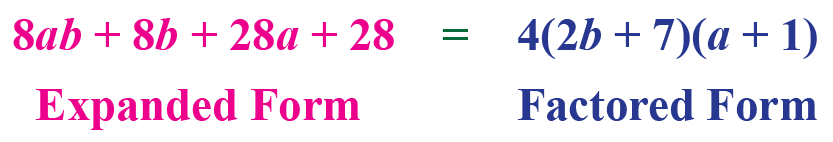## What Are the Types of Factoring Polynomials?

Let's discuss a few methods of factoring polynomials.

### Method of Common Factors

Consider a simple example: $$3x+9$$

By factorizing each term we get, $$(3\times x)+(3 \times 3)$$

By distributive law, $$3x+9=(3\times x)+(3 \times 3)=3(x+3)$$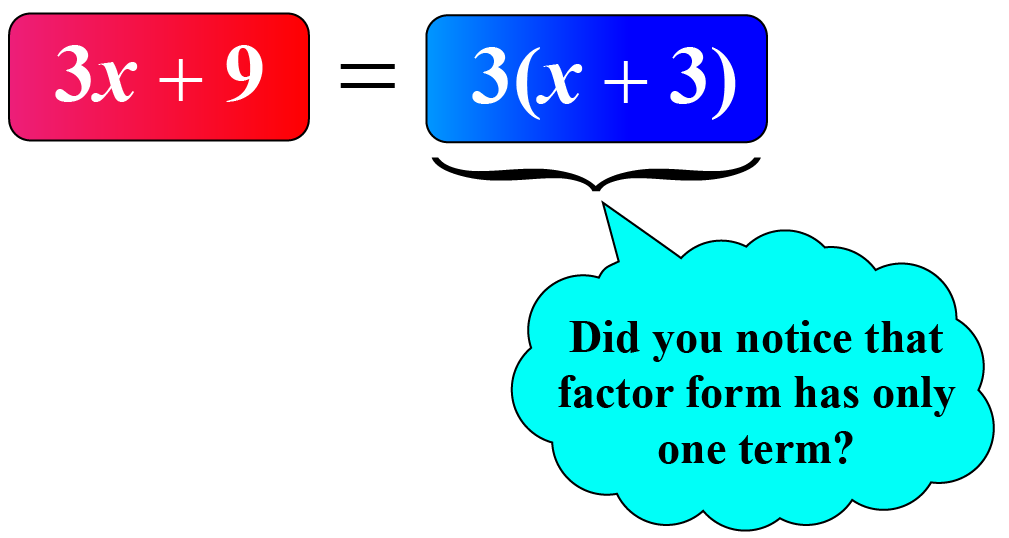### Factoring Polynomials By Grouping

The method of grouping is already explained in the previous section

The polynomial is factorized as $$8ab+8b+28a+28=4(2b+7)(a+1)$$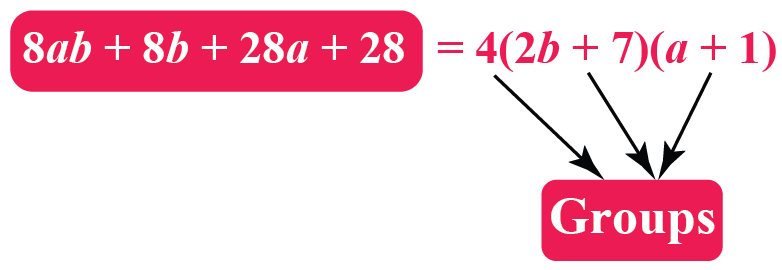### Method Using Algebraic Identities

Remember a few identities that are used for factorization.

 $$(a+b)^2=a^2+b^2+2ab$$
 $$(a-b)^2=a^2+b^2-2ab$$
 $$(a+b)(a-b)=a^2-b^2$$

Let's factorize the polynomial $$4z^{2}-12z+9$$

Observe that $$4z^{2}=(2z)^{2}$$, $$12z=2\times 3\times 2z$$, and $$9=3^{2}$$

So, we can write $$4z^{2}-12z+9=(2z)^{2}-2\times 2z\times 3+3^{2}$$
$$=(2z-3)^2$$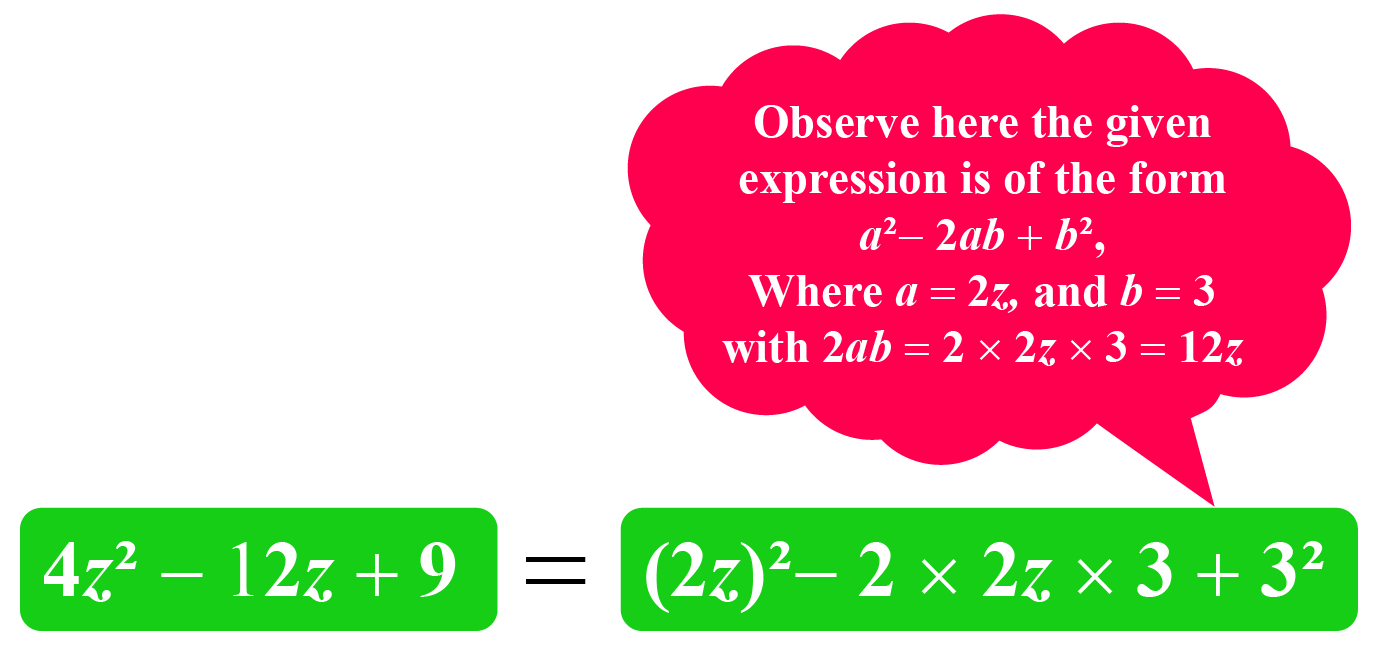## Factoring Polynomials Calculator

Arrange the variables and constants to form a rectangle and find factors of the given polynomial.

Drag the red dots to move the boxes.

We hope factoring polynomials calculator served you really well!

## Solved Examples

 Example 1

Carlos will begin attending classes for the sixth grade from next week.

He suddenly realized that he doesn't have either a notebook or a pen.

He goes to a shop to purchase them and realizes that the cost of a notebook is twice more than $4 for a pen.Represent this above information using a polynomial. Can you find the factors of the polynomial? Solution Let's assume the following. The cost of a pen =$$$x$$

According to the given information, the cost of the notebook can be expressed as $$2x + 4$$

2 is a common factors in the polnomial $$2x + 4$$.

 $$\therefore$$ The factors $$2x + 4$$ are 2 and $$x+2$$.
 Example 2

Emma asked Jolly to factorize the polynomial $$6xy-4y+6-9x$$

Jolly wants to factorize it using the method of regrouping.Can you help them?

Solution

We observe that we have no common factor among all the terms in the expression $$6xy-4y+6-9x$$

Let's deal with $$6xy-4y$$ and $$6-9x$$ separately.

\begin{align}6xy-4y&=2y(3x-2)\\6-9x&=-3(3x-2)\end{align}

So, the given expression can be written as

\begin{align}6xy-4y+6-9x&=(2y-3)(3x-2)\end{align}

 $$\therefore$$ Factors of $$6xy-4y+6-9x$$ are $$(2y-3)$$ and $$(3x-2)$$
 Example 3

Jake is stuck with one question in his maths assignment.The question is on factoring cubic polynomials $$x^3+5x^2+6x$$

Can you help him?

Solution

Notice that $$x$$ is a common factor in $$x^3+5x^2+6x$$.

So, $$x^3+5x^2+6x=x(x^2+5x+6)$$

We’ll factor $$x^2+5x+6$$ in two binomials, namely $$(x+a)$$ and $$(x+b)$$.

Expand $$(x+a)(x+b)$$.

\begin{align}(x+a)(x+b)&=x(x+b)+a(x+b)\\&=x^2+bx+ax+ab\\&=x^2+(a+b)x+ab\end{align}

Let’s try to find $$a$$ and $$b$$ such that $$(a+b)$$ is mapped to 5 and $$ab$$ is mapped to 6.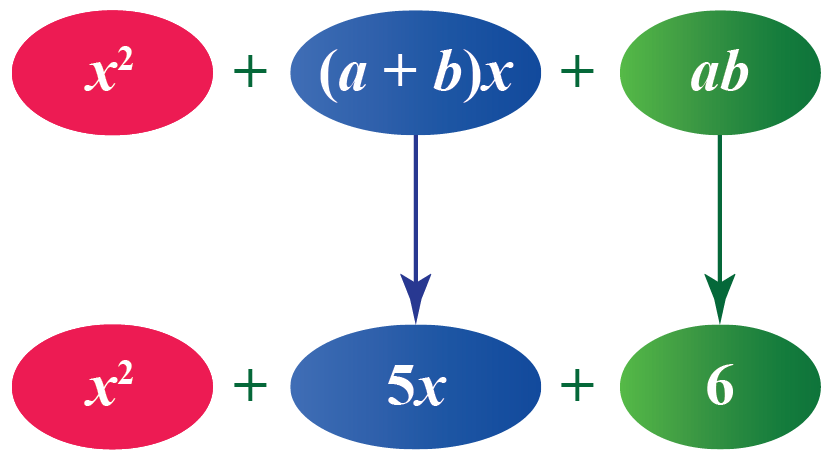The factors of $$6$$ are $$1$$, $$2$$, $$3$$ and $$6$$. Find the pairs $$a$$ and $$b$$ from $$1$$, $$2$$, $$3$$ and $$6$$ such that $$a+b=5$$ and $$ab=6$$

This is the right pair: $$2$$ and $$3$$, because, $$2+3 = 5$$ and $$2 \times 3=6$$

Thus, the cubic polynomial can be factored as $$x(x+2)(x+3)$$.

 $$\therefore$$ $$x^3+5x^2+6x=x(x+2)(x+3)$$

Sometimes working with a hard polynomial is difficult. But don’t worry!

Here are a few tips and tricks for you to follow while factorization.Tips and Tricks
1. Check out for any common terms in a polynomial and take the greatest common factor.
2. Check if any algebraic identities are applicable in the polynomial.
3. Keep factoring the polynomial until you reach the simplest form, that is, the form that is not further divisible.

## Interactive Questions

Here are a few activities for you to practice.

## Let's Summarize

The mini-lesson targeted the fascinating concept of Trigonometric Ratios. The math journey around Trigonometric Ratios starts with what a student already knows, and goes on to creatively crafting a fresh concept in the young minds. Done in a way that not only it is relatable and easy to grasp, but also will stay with them forever. Here lies the magic with Cuemath.

At Cuemath, our team of math experts is dedicated to making learning fun for our favorite readers, the students!

Through an interactive and engaging learning-teaching-learning approach, the teachers explore all angles of a topic.

Be it worksheets, online classes, doubt sessions, or any other form of relation, it’s the logical thinking and smart learning approach that we, at Cuemath, believe in.

## FAQs on Graphing Complex Numbers

### 1. How do you find the factors of a polynomial?

To write a polynomial in factored form, it must be expressed as a product of terms in its simplest form.

The terms could be constant or linear or any polynomial form which is not further divisible.

### 2. What is the meaning of factoring polynomials by grouping?

Factoring polynomials by grouping means to factorize the polynomial by the method of grouping that allows us to rearrange the terms of the expression that leads to factorization.

### 3. How do you do the prime factorization of polynomials?

Use the methods mentioned below to find the prime factorization of polynomials.

1. Method of Common Factors
2. Method of Grouping
3. Method Using Algebraic Identities

### 4. What are the 3 types of factoring polynomials?

The 3 types of factoring polynomials are:

1. Method of Common Factors
2. Method of Grouping
3. Method Using Algebraic Identities

### 5. How do you find the factors a polynomial with 5 terms?

The following steps show how to factor a polynomial with 5 terms.

1. Write the polynomial in the standard form.
2. Take the greatest common factor out if it exists.
3. Try to find at least 3 roots of the polynomial. If $$\alpha$$ is a root of the polynomial, then $$x-\alpha$$ is a factor of the polynomial.
4. After finding the 3 linear factors, we are left with a quadratic polynomial. Find the product of the leading coefficient and the constant term.
5. Determine the factors of the product found in step 3 and check which factor pair will result in the coefficient of $$x$$.
6. After choosing the appropriate factor pair, keep the sign in each number such that while operating them we get the result as the coefficient of $$x$$ and on finding their product the number is equal to the number found in step 3.
7. Now, you have 4 terms in the expression and so we use the method of regrouping to factorize.

### 6. What are the four methods of factoring polynomials?

The four methods of factoring polynomials are:

1. Method of Common Factors
2. Method of Grouping
3. Method Using Algebraic Identities
4. Method of Finding Roots

More Important Topics
Numbers
Algebra
Geometry
Measurement
Money
Data
Trigonometry
Calculus